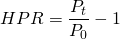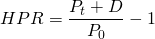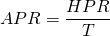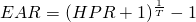# Return calculation

Calculating returns is a key aspect of investing. At the same time, we should keep in mind that returns are only half the equation. In addition to a security’s return, investors should always take into consideration the security’s risk as well. A good understanding of both is indispensable. In what follows, we will explain how to calculate and use holding period returns, annual percentage rates, and effective annual rates. Investors should be aware of the return on their investments and should be able to calculate returns themselves.

## Holding period return

Holding period returns (HPR) are the most basic and easiest to calculate returns. They are calculated by simply dividing the terminal value of an investment (Pt) by the initial investment value (P0) and subtracting 1. Obviously, the holding period return measures the percentage gain in wealth over the investment horizon.In case the investor received income, dividends, or interest over the investment horizon, the holding period return formula just expands by adding this income (denoted D) in the numerator.## Annual percentage rate

Unfortunately, one disadvantage of holding period returns is that returns on assets with different investment horizons can’t be compared. To do this, we need to divide the holding period returns by their respective  investment horizon T.  The annual percentage rate (APR) we obtain measures the yearly return on each asset.## Effective annual rate

Unfortunately, the annual percentage rate does not take interest compounding into account. Therefore, we need to adjust the HPR again. This slightly more complicated formula is shown below. The effective annual rate (EAR) measures the annual rate of return on an asset, taking into account any potential interest compounding. It is the most complicated way of calculating a security’s return, but probably also the most accurate.## Summary

The return on investment of a security can be calculated in different ways. and investors should be wary of the the advantages and disadvantagese of different methods. Misrepresentation of returns is a way of misleading uninformed investors. Holding period returns, annual percentage rates, and effective annual rates are among the most important ways of calculating returns.

### Return calculation

Need to have more insights? Download our free excel file: return calculation.